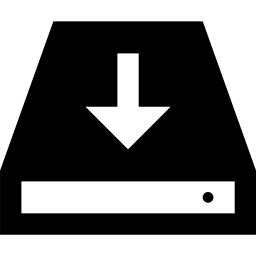## Learn to Use ITensor

main / formulas / excited_dmrg C++v3 | C++v2 | Julia

# Compute excited states with DMRG

### Example code:

#include "itensor/all.h"

using namespace itensor;

int
main()
{
int N = 100;

//
// Initialize the site degrees of freedom.
//
auto sites = SpinHalf(N,{"ConserveQNs=",false}); //make a chain of N spin 1/2's

//Transverse field
Real h = 4.0;

//
// Use the AutoMPO feature to create the
// transverse field Ising model
//
// Factors of 4 and 2 are to rescale
// spin operators into Pauli matrices
//
auto ampo = AutoMPO(sites);
for(int j = 1; j < N; ++j)
{
ampo += -4,"Sz",j,"Sz",j+1;
}
for(int j = 1; j <= N; ++j)
{
ampo += -2*h,"Sx",j;
}
auto H = toMPO(ampo);

//
// Set the parameters controlling the accuracy of the DMRG
// calculation for each DMRG sweep.
//
auto sweeps = Sweeps(30);
sweeps.maxdim() = 10,20,100,100,200;
sweeps.cutoff() = 1E-10;
sweeps.niter() = 2;
sweeps.noise() = 1E-7,1E-8,0.0;
println(sweeps);

//
// Begin the DMRG calculation
// for the ground state
//
auto [en0,psi0] = dmrg(H,randomMPS(sites),sweeps,{"Quiet=",true});

println("\n----------------------\n");

//
// Make a vector of previous wavefunctions;
// code will penalize future wavefunctions
// for having any overlap with these
//
auto wfs = std::vector<MPS>(1);
wfs.at(0) = psi0;

//
// Here the Weight option sets the energy penalty for
// psi1 having any overlap with psi0
//
auto [en1,psi1] = dmrg(H,wfs,randomMPS(sites),sweeps,{"Quiet=",true,"Weight=",20.0});

//
// Print the final energies reported by DMRG
//
printfln("\nGround State Energy = %.10f",en0);
printfln("\nExcited State Energy = %.10f",en1);

//
// The expected gap of the transverse field Ising
// model is given by Eg = 2*|h-1|
//
// (The DMRG gap will have finite-size corrections.)
//
printfln("\nDMRG energy gap = %.10f",en1-en0);
printfln("\nTheoretical gap = %.10f",2*std::fabs(h-1));

//
// The overlap <psi0|psi1> should be very close to zero
//
printfln("\nOverlap <psi0|psi1> = %.2E",inner(psi0,psi1));

return 0;
}Download the example codeBack to FormulasBack to Main